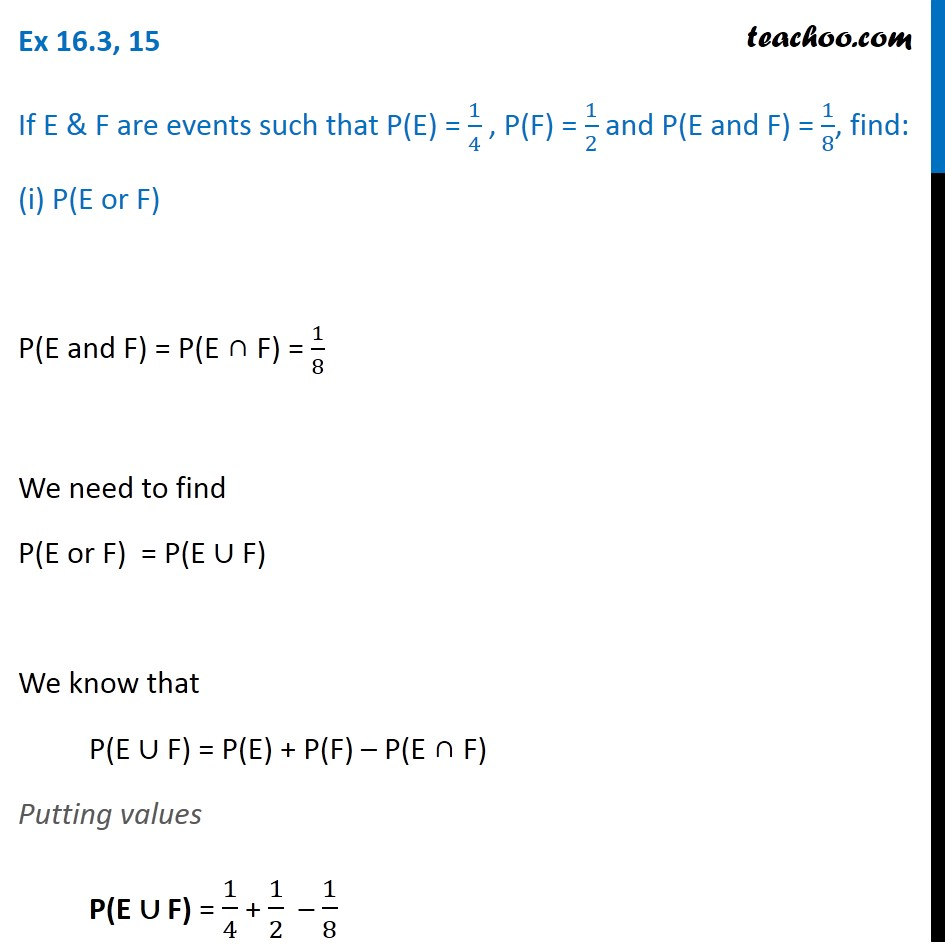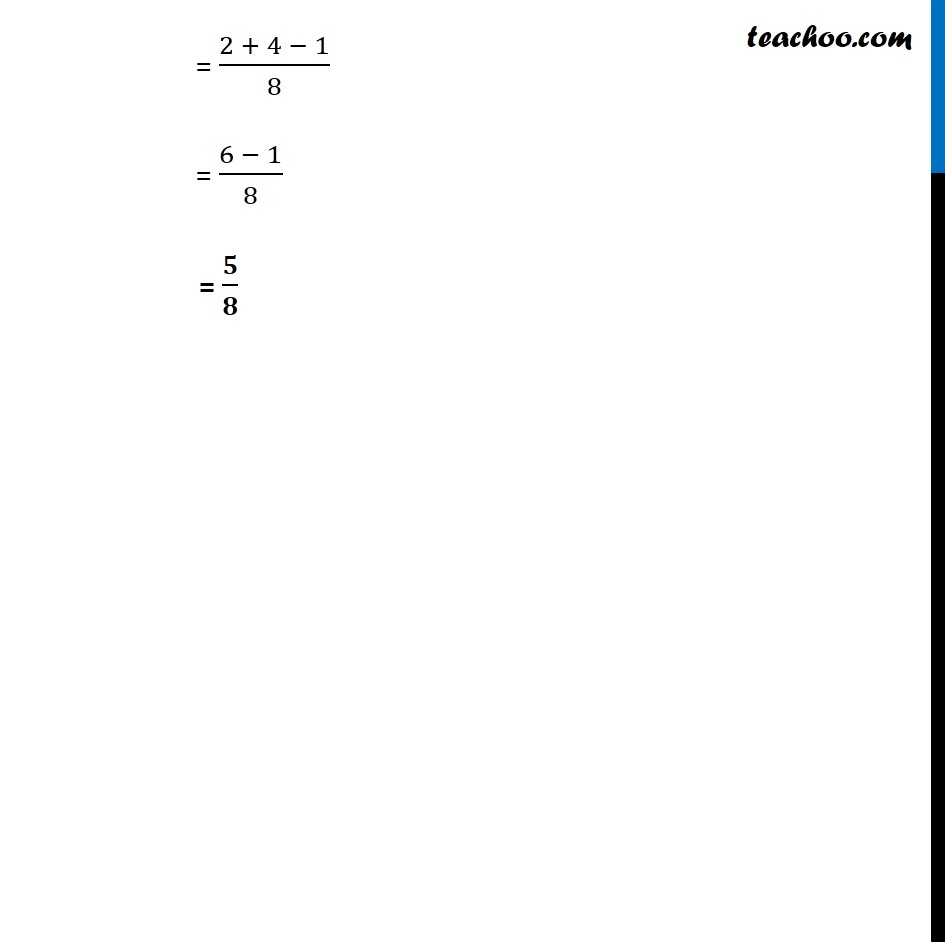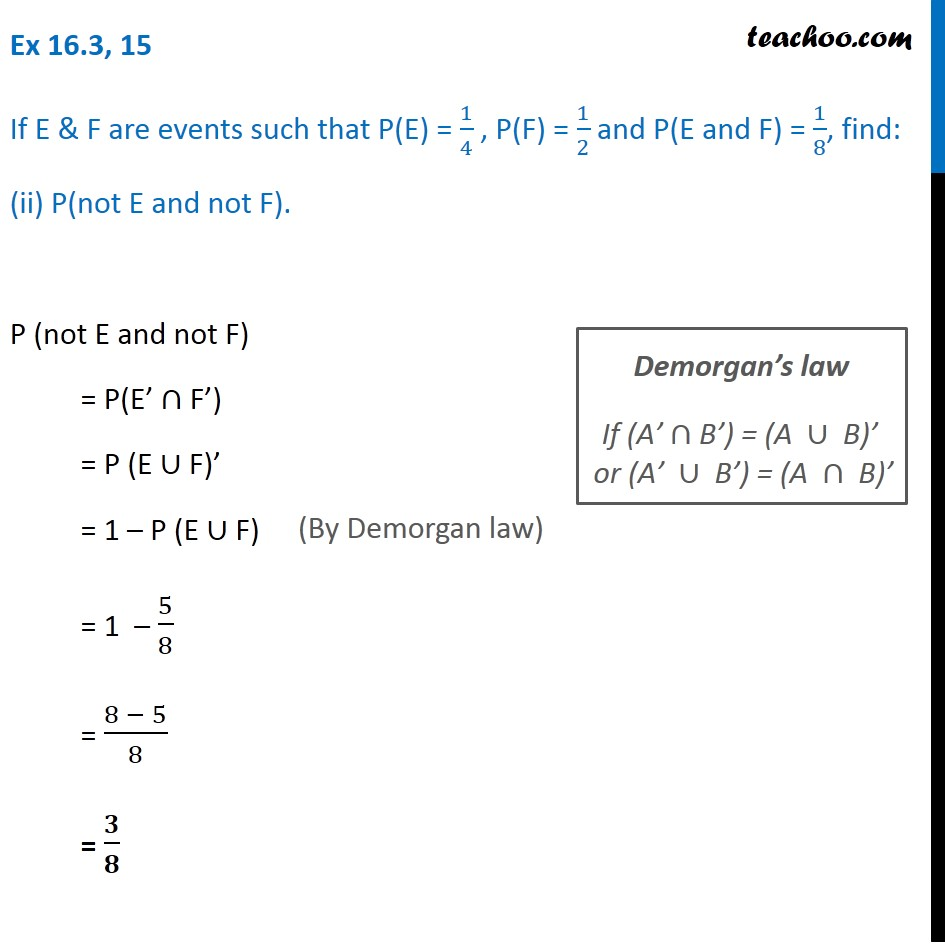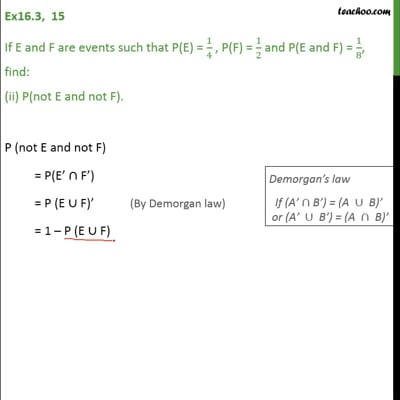Ex 16.3

Chapter 16 Class 11 Probability
Serial order wiseThis video is only available for Teachoo black users

Maths Crash Course - Live lectures + all videos + Real time Doubt solving!

### Transcript

Ex 16.3, 15 If E & F are events such that P(E) = 1/4 , P(F) = 1/2 and P(E and F) = 1/8, find: (i) P(E or F) P(E and F) = P(E ∩ F) = 1/8 We need to find P(E or F) = P(E ∪ F) We know that P(E ∪ F) = P(E) + P(F) – P(E ∩ F) Putting values P(E ∪ F) = 1/4 + 1/2 – 1/8 = (2 + 4 − 1)/8 = (6 − 1)/8 = 𝟓/𝟖 Ex 16.3, 15 If E & F are events such that P(E) = 1/4 , P(F) = 1/2 and P(E and F) = 1/8, find: (ii) P(not E and not F). P (not E and not F) = P(E’ ∩ F’) = P (E ∪ F)’ = 1 – P (E ∪ F) = 1 – 5/8 = (8 − 5)/8 = 𝟑/𝟖 Demorgan’s law █("If (A’" ∩"B’) = (A " ∪" B)’ " @"or (A’ " ∪" B’) = (A " ∩" B)’" )